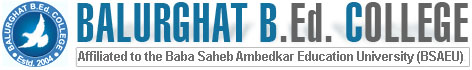Library AQAR IQAC ALUMINI Collaboration RTI Magazine

03522 - 271515/ 259179    balurbedcollege@gmail.com

#### Detials PDF

Details of Rooms Size Number

Class Room

Class Room

52.87 Sq.mtrs x 3      = 158.61 Sq.mtrs

29.54 Sq.mtrs x 8      = 236.32 Sq.mtrs

3

8

Class Room

40.64 Sq.mtrs x 1      =40.64 Sq.mtrs

1

Class Room

85.56 Sq.mtrs x 1      = 85.56 Sq.mtrs

1

Class Room

65.50 Sq.mtrs x 1      = 65.50 Sq.mtrs

1

Class Room

44.21 sq. mtrs x 1      = 44.21 sq.mtrs

1

Class Room

29.54 Sq.mtrs x 1      = 29.54sq.mtrs

1

Class Room

53.30 Sq.mtrs x 14    = 746.27 sq.mtrs.

14

Class Room

42.25 Sq.mtrs x 2      =84.50 sq.mtrs.

2

Class Room

42.27 Sq.mtrs x 2      =84.54 sq.mtrs

2

Class Room

30.00 Sq.mtrs x 1      = 30.00 sq.mtrs.

1

Class Room

54.90 Sq.mtrs x 2      = 109.80 sq.mtrs

2

Class Room

57.60 Sq.mtrs x 2       =115.20sq.mtrs

2

Class Room

54.00 Sq.mtrs x 1       =54.00 sq.mtrs

1

Class Room

57.90 Sq.mtrs x 1       =57.90sq.mtrs

1

Details of Rooms Size Number

Multipurpose Hall

Multipurpose Hall

(ii) Multipurpose Room

(Language Lab & English Class Room)

(iii) Multipurpose Room

(iv) Multipurpose Room

(use for Workshop/Seminar)

(v) Any other Room

Computer Science Lab-

Geography Lab-

141.80 Sq.mtrs. x 1     =141.80 Sq.mtrs

226.95 Sq.mtrs. x 1     =226.90 Sq.mtrs

55.34 Sq.mtrs. x 1      =55.34 Sq.mtrs.

44.21 Sq.mtrs x 6       =265.29 Sq.mtrs.

90.00 Sq.mtrs. x 1      =90.00 Sq.mtrs.

79.34 Sq.mtrs x 5       = 398.70 Sq.mtrs

40.64 Sq.mtrs x 1       = 40.64 Sq.mtrs

40.64 Sq.mtrs x 1       = 40.64 Sq.mtrs

1

1

1

6

1

5

1

1

Details of Rooms Size Number

Principal?s Room

26.87 Sq.mtrs x 1      = 26.87 Sq.mtrs

16.00 Sq.mtrs  x 1     = 16.00 Sq.mtrs

16.12 Sq.mtrs x 1      = 16.12 Sq.mtrs

1

1

1

Teachers? Room

20.00 Sq.mtrs x 1       = 20.00 Sq.mtrs

44.21 Sq.mtrs x 1        = 44.21 Sq.mtrs

1

1

Science & Mathematics resource centre Laboratory (Physics, chemistry, Biology)

40.64 Sq.mtrs x 3       =121.92 Sq.mtrs

40.86 Sq.mtrs x 1        = 40.86 Sq.mtrs

3

1

Psychology resource centre/Lab

40.64 Sq.mtrs x 1   =40.64 Sq.mtrs

1

ICT Resource Centre (SUPW) Work Education

30.16 Sq.mtrs x 1         = 30.16 Sq.mtrs

1

Art and Craft Resource Centre

53.30 Sq.mtrs x 1     = 53.30 Sq.mtrs

1

Any Other Room (Health and Physical Education Resource Centre)

121.92 Sq.mtrs x 1    =121.92 Sq.mtrs

1

44.21 Sq.mtrs x 1      =44.21 Sq.mtrs

79.74 Sq.mtrs x 1      = 79.74 Sq.mtrs

1

1

Any other room (Visiting Room cum Management)

44.21 Sq.mtrs x 1      =44.21 Sq.mtrs

1

Any other Room

(ii) Any other Room (Music)

(iii) Any other Room

26.45 Sq.mtrs x 1      =26.45 Sq.mtrs

29.04 Sq.mtrs x 1       =29.04 Sq.mtrs

141.80 Sq.mtrs x 1     =141.80 Sq.mtrs

1

1

1

Tutorial/Seminar Room

85.56 Sq.mtrs x 1         =85.56 Sq.mtrs

1

Canteen

28.86 Sq.mtrs x 1        =28.86 Sq.mtrs

1

Separate Toilet Facility for Boys and Girls

2 Boys-88.48 + 7.29 Sq.mtrs=95.77 Sq.mtrs

3 Girls-4.16 x 2+7.29Sq.mtrs =15.61Sq.mtrs

16.70 Sq.mtrs x 1       = 16.70 Sq.mtrs

18.26 Sq.mtrs x 1        = 18.26 Sq.mtrs

Total=146.34 Sq.mtrs

2

3

1

1

Any other facilities (Parking space/Cycle Stand)

(ii) Garage

136.51 Sq.mtrs x 1 =136.51 Sq.mtrs

91.88 Sq.mtrs x 1    =91.88 Sq.mtrs

1

1

Store Room

22.94 Sq.mtrs

06.51 Sq.mtrs

16.12 Sq.mtrs (sports)

53.30 Sq.mtrs

45.59 Sq.mtrs                         Total=144.46 Sq.mtrs

1

1

1

1

1

Multipurpose Hall (Auditorium cum multipurpose Class Room)

190.96 Sq.mtrs       =190.96 Sq.mtrs

1

Boys Common Room

Girls Common Room

59.04 Sq.mtrs              = 59.04 Sq.mtrs

38.50 Sq.mtrs                = 38.50 Sq.mtrs

1

1

Library

111.45 Sq.mtrs       = 111.45 Sq.mtrs

1

Total Build up area for B.Ed. Block        =   2616.63 Sq.mtrs

For D.El.Ed. Block  =   1434.00 Sq.mtrs

For M.Ed. Block      =   1758.66 Sq. mtrs___________

Total  =   5809.29 Sq. mtrs